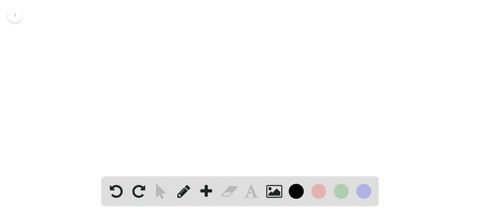### Evaluate each iterated integral. (Many of these u…

06:35Georgia Southern University
Problem 10

Evaluate each integral.
$$\int_{1}^{5} y e^{4 x+y^{2}} d x$$

$=4 y\left(e^{y^{2}+20}-e^{y^{2}+4}\right)$

## Discussion

You must be signed in to discuss.

## Video Transcript

okay. And a girl from one to five. Of Why? To the four x plus Why squared Teo? Yeah, actually, D x. Okay, so yeah, this why we're treating as a constant so we can actually factor that out. And we're just looking for an anti derivative of e to the forex. That's why squared with respect X, That's not too bad. It's just going to be itself. But we're going to divide by a factor of four to account for the changeable. Why ever for and then need to the forex. Plus why Squared would get us verify this by taking derivative We wanted to and evaluation from one to five feet. Why, whatever for time's he to the four kinds five. Twenty. That's why I squared minus me too. The four times one plus y squared